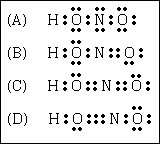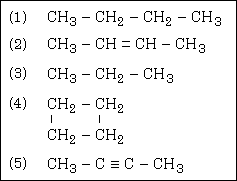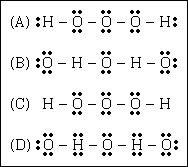MainTutorialsOrganic ChemistryPractice TestsOnline QuizzesReference ToolsU.S. National Chemistry Olympiad: 1988 Local Section Test

1. The recommended adult dose of Elixophyllin, a drug used to treat asthma, is 6.0 mg per kg of body weight. Calculate the dose, in milligram, for a 170-pound person.

(A) 3.5 x 10Ż2 mg
(B) 460 mg
(C) 1020 mg
(D) 2250 mg

2. Expressed to the appropriate number of significant figures, the result of the calculation [(5.031-4.96)(2.38)] ÷ 3.91

(A) 0.04
(B) 0.043
(C) 0.0432
(D) 0.04322

3. Using the cathode-ray tube, J.J. Thompson was able to determine

(A) the charge of an electron
(B) the charge-to-mass ratio of an electron
(C) the charge-to-mass ratio of a proton
(D) that the nucleus of an atom is very small but contains most of the mass

4. The partial symbol for a particular ion is 24Mg2+. The number of electrons contained is one of these ions is

(A) 2
(B) 10
(C) 12
(D) 22

5. The mass percent of carbon in methanol, CH3OH, is

(A) 12.0%
(B) 12.5%
(C) 16.7%
(D) 37.5%

6. The correct molecular formula of a compound that has an empirical formula C3H4O and a molecular mass of 168 grams/mole is

(A) C3H4O
(B) C6H8O2
(C) C8H8O4
(D) C9H12O3

7. A gas sample is contained in a flask connected to a U-shaped manometer. Measured from the bottom of the U-tube, the height of the mercury on the side connected to the flask is 62 mm. The height of the mercury on the side open to the atmosphere is 182 mm. The atmospheric pressure is measured with a barometer to be 759 mm Hg. The pressure of the gas in the container is

(A) 120 mm Hg
(B) 639 mm Hg
(C) 821 mm Hg
(D) 879 mm Hg

8. A sample of a gas with volume of 800 mL and pressure of 1.0 atm is transferred to a second container which has a volume of 250 mL and is at the same temperature. The pressure of the gas in the new container is

(A) 0.31 atm
(B) 1.0 atm
(C) 1.5 atm
(D) 3.2 atm

9. A 1.22 gram of an unknown volatile substance is vaporized at 100°C is 0.993 atm. The molecular weight of the substance is

(A) 49.2 g/mol
(B) 122 g/mol
(C) 183 g/mol
(D) 308 g/mol

10. Iron(III) oxide can be reduced with carbon monoxide to form metallic iron as described by the unbalanced chemical equation

Fe2O3 + CO ---> Fe + CO2

The number of moles of CO required to form one mole of Fe from its oxide is

(A) 1
(B) 1.5
(C) 2
(D) 3

11. A product of the reaction of silver nitrate, AgNO3, with calcium chromate, CaCrO4, in aqueous solution is

(A) CaNO3
(B) Ag2NO3
(C) AgCrO4
(D) Ag2CrO4

12. Sodium nitrate, heated in the presence of an excess of hydrogen, forms water according to the two-step process

 2 NaNO3 ---> 2 NaNO2 + O2 2 H2 + O2 ---> 2 H2O

How many grams of sodium nitrate are required to form 9 grams of water?

(A) 21.3
(B) 42.5
(C) 69.0
(D) 85.0

13. Which quantity of nickel has the largest mass?

(A) one mole
(B) 6.02 x 1023 atoms
(C) 58.7 grams
(D) 22.4 moles

14. Which element should have properties most like those of phosphorus?

(A) Si
(B) S
(C) As
(D) Sb

15. Which of the following isoelectronic ions should have the smalles ionic radius?

(A) Sc3+
(B) Ca2+
(C) K+
(D) ClŻ

16. Which element has no known stable chemical compounds?

(A) Au
(B) Ne
(C) Pt
(D) Xe

17. For which pair of atoms is the electronegativity difference the greatest?

(A) B, C
(B) Li, I
(C) K, Cl
(D) Se, S

18. In which molecule would you find a triple covalent bond?

(A) O2
(B) N2
(C) O3
(D) FeCl3

19. How many unpaired electrons are found in the most stable electronic state of a sulfur atom?

(A) 0
(B) 2
(C) 4
(D) 6

20. The arrangement of the atoms in the ammonia molecule at its lowest energy is described as

(A) trigonal pyramid
(B) trigonal planar
(C) trigonal bipyramid
(D) square planar

21. The most reasonable Lewis structure for the HNO2 molecule is22. In which electronic state would a hydrogen atom be able to absorb a photon of elctromagnetic radiation but not be able to emit a photon?

(A) 1s1
(B) 2s1
(C) 3p1
(D) 4d1

23. Which molecule is non-polar?

(A) SO3
(B) SO2
(C) CO
(D) H2O

24. Which compound is most likely to be ionic?

(A) GaAs
(B) ScBr3
(C) NO2
(D) ClO2

25. The very high heat of vaporization of H2O is mainly a result of

(A) van der Waals forces
(B) covalent bonds
(C) interionic attractions
(D) hydrogen bonding

26. A standard solution of 0.154 M HCl is used to determine the concentration of a NaOH solution whose concentration is unknown. If 33.5 mL of the acid solution are required to neutralize 25.0 mL of the base solution, the concentration of the NaOH in moles/liter is

(A) 25.0 / (0.154)(33.5)
(B) (0.154)(25.0) / 33.5
(C) (33.5 + 25.0) / 0.514
(D) (0.514)(33.5) / 25.0

27. A species which acts as an acid according to the Bronsted model of acids and bases is

(A) O2Ż
(B) NH4+
(C) CH4
(D) NH2Ż

28. The hydrogen ion concentration corresponding to a pH of 8.64 is

(A) 5.36
(B) 4.4 x 10Ż8
(C) 4.4 x 10Ż9
(D) 2.3 x 10Ż9

29. Consider a 1.0 M solution of each of the following salts. Which will have the lowest freezing point?

(A) NaCl
(B) BaCl2
(C) Na2SO4
(D) Al(NO3)3

30. Which has the greatest molar solubility in water at 25°C?

(A) ammonium nitrate
(B) calcium sulfate
(C) copper (II) sulfide
(D) silver carbonate

31. The heat of combustion of CH4 is 882 kJ/mole. How much heat would be liberated by the complete combustion of 4.00 g of CH4?

(A) 5.5 kJ
(B) 220 kJ
(C) 882 kJ
(D) 3530 kJ

32. Which process is exothermic?

(A) Water freezes to produce ice
(B) Ether evaporates to form vapor
(C) Dry ice sublimes to form gas
(D) Water boils to produce steam

33. The normal boiling point of a liquid is defined as:

(A) the pressure at which a liquid vaporizes
(B) the temperature at which a liquid vaporizes
(C) the temperature at which the vapor pressure of a liquid equals 1 atm
(D) the temperature at which the vapor pressure of a liquid equals the barometric pressure

34. In the reaction

SO2 + 2 H2S ---> 3 S + 2 H2O

(A) sulfur is oxidized and hydrogen is reduced
(B) sulfur is reduced and there is no oxidation
(C) sulfur is reduced and hydrogen is oxidized
(D) sulfur is both reduced and oxidized

35. Which group among the representative (main-group) elements contains the most powerful oxidizing agent?

(A) group I
(B) group III
(C) group VI
(D) group VII

36. The following standard electrode (reduction) potentials refer to aqueous solution at 25°C.

 Ni2+(aq) + 2eŻ <===> Ni(s) E° = - 0.25 V Cu2+(aq) + 2eŻ <===> Cu(s) E° = + 0.34 V Fe3+(aq) + eŻ <===> Fe2+(aq) E° = + 0.77 V

What is the standard potential for the reaction

Cu2+(aq) + Ni(s) <===> Cu(s) + Ni2+(aq) ?

(A) 0.09 V
(B) 0.59 V
(C) 0.86 V
(D) 1.02 V

37. Which ion, in solution, can be oxidized by appropriate chemical means but also can be reduced by a different chemical reaction?

(A) Fe2+
(B) FŻ
(C) CO32Ż
(D) NO3Ż

38. Choose the pair of salts whose aqueous solutions would form a precipitate upon mixing.

(A) NaNO3 and MgBr2
(B) KNO3 and (NH4)2CO3
(C) BaCl2 and K2CO3
(D) Na2SO4 and (NH4)2S

39. Which statement desribing chemical equilibrium is NOT correct?

(A) A system at chemical equilibrium has a constant mass.
(B) A system in chemical equilibrium acts so as to oppose slight disturbances.
(C) Forward and reverse reactions proceed at the same rate in a system at chemical equilibrium.
(D) Reactant and product concentrations vary with time in a system at chemical equilibrium.

40. The equilibrium constant for the process

Ag2S (s) + H2O (l) <===> 2 Ag+ (aq) + S2Ż (aq)

has a very small numerical value. This implies that

(A) silver sulfide reacts extensively with water.
(B) the solubility of silver sulfide in water is very small.
(C) solutions can be prepared containing large concentrations of silver ions and sulfide ions.
(D) the process described by the equation is not affected by temperature.

41. The rate of a chemical reaction between substances A and B is found to follow the rate equation

rate = k[A]2[B]

where k is a constant. If the concentration of A is halved, what should be done to the concentration of B to make the reaction go at the same rate as before?

(A) The concentration of B should be kept constant.
(B) The concentration of B should be doubled.
(C) The concentration of B should be halved.
(D) The concentration of B should be quadrupled.

42. If the average velocity of SO2 molecules at 25 °C is 0.2 mile/sec., what is the average velocity of CH4 molecules in miles/sec. at the same temperature?

(A) 0.1
(B) 0.2
(C) 0.4
(D) 0.8

43. Cesium-137 decays spontaneously to emit beta particles and form Barium-137. Its half-life is 30 years. Approximately what length of time will have elapsed before 97% of the Cs-137 in a particular sample will have decomposed?

(A) 20 years
(B) 60 years
(C) 150 years
(D) 2900 years

44. An alpha particle is

(A) a charged hydrogen atom.
(B) a negatively charged particle of mass number one.
(C) an electron.
(D) the nucleus of a helium atom.

45. At standard temperature and pressure, chlorine is

(A) a white, unstable solid.
(B) a brown liquid
(C) a soft, reactive solid.
(D) a yellow-green gas.

46. Which has the greatest electrical conductivity at room temperature?

(A) pure water
(B) 0.010 M aqueous sodium chloride solution.
(C) 0.010 M aqueous sucrose solution.
(D) pure silver

47. Which substance will react appreciably with water, at room temperature and pressure, to produce hydrogen?

(A) H2S
(B) KH
(C) HCl
(D) NH3

48. How many grams of SO2 could be formed if a mixture of 128 g of sulfur and 100 g of oxygen are mixed and ignited?

(A) 100 g
(B) 114 g
(C) 200 g
(D) 228 g

49. A compound was found to contain 21.6% Mg, 21.4% C, and 57.0% by mass. What is the simplest formula for this compound?

(A) MgCO3
(B) MgC2O4
(C) Mg2CO3
(D) Mg(CO)4

50. Consider the hydrocarbons identified by the numbers 1 through 5 below:Which pair of the above compounds are isomers?

(A) 1 and 4
(B) 1 and 3
(C) 2 and 4
(D) 2 and 5

51. Consider the reaction at equilibrium:

2 SO2(g) + O2(g) <===> 2 SO3(g) DH = -7.8 kcal.

Which procedure would result in the formation of more SO2?

(C) an increase in pressure at constant temperature
(D) a decrease in temperature at constant pressure

52. Formulas of five compunds of element Z are listed below. Which one of them must be incorrect?

(A) Z2O3
(B) Z2(SO4)3
(C) ZPO4
(D) Z2Cl3

53. The oxidation number for sulfur in K2SO3 is

(A) -1
(B) +3
(C) +4
(D) +6

54. Given the following bond energies:

 C -- H 414 kJ / mol C -- Cl 339 kJ / mol Cl -- Cl 243 kJ / mol H -- Cl 432 kJ / mol

How much energy would be released in the reaction

CH4(g) + 2 Cl2(g) ---> CH2Cl2(g) + 2 HCl(g) ?

(A) 150 kJ
(B) 150 kJ
(C) 228 kJ
(D) 571 kJ

55. The electron configuration for Mn2+ is

(A) [Ar] 4s2 3d3
(B) [Ar] 3d5
(C) [Ar] 4s1 3d5
(D) [Ar] 4s1 3d4

56. The energy of electromagnetic radiation is greater if

(A) its wavelength is short and its frequency is high.
(B) its wavelength is short and its frequeny is low.
(C) its wavelength is long and its frequency is low.
(D) its wavelength is long and its frequency is high.

57. Hydrogen trioxide, H2O3, is an unstable compound but can be isolated in small quantities. Which is a reasonable Lewis structure for this molecule?58. What kind of ions have, in general, the strongest interaction with water molecules in aqueous solution?

(A) ions with large electric charge and larger diameter
(B) ions with small electric charge and small diameter
(C) ions with large electrid charge and small diameter
(D) ions with small electric charge and large diameter

59. What is the molarity of acid in vinegar containing 4.0% acetic acid by weight and having a density of 1.02 g/mL?

(A) 0.50 M
(B) 0.68 M
(C) 0.75 M
(D) 1.36 M

60. If the smallest whole-number coefficients are used to balance the oxidation-reduction reaction

Cr2O3 + Na2CO3 + KNO3 ---> Na2CrO4 + CO2+ KNO2

the sum of the coefficients in the balanced equation is

(A) 13
(B) 14
(C) 14
(D) 19

61. The standard enthalpies of formation for two oxides of nitrogen are:

 NO2(g) DH°f = 32 kJ / mole NO(g) DH°f = 90 kJ / mole

For the reaction 2 NO2(g) ---> 2 NO(g) + O2(g) calculate D

(A) -122 kJ
(B) 58 kJ
(C) 116 kJ
(D) 122 kJ

62. Which molecules would be expected to have a dipole moment?

1. SOCl2
2. ICl
3. FCl
4. BCl3

(A) 1 and 2
(B) 3
(C) 1, 2, and 3
(D) all of them

63. A sample of CaCO3 was placed in an evacuated oven 5.34 liters in volume and heated to 900 C. A pressure of 69.7 mm Hg was observed as a result of the complete decomposition of the sample to CaO and CO2.

What was the weight of CaCO3 that was originally placed in the oven?

(A) [(100) (69.7) (5.34)] ÷ [(0.082) (900)]
(B) [(69.7) (273) (5.43)] (0.082) ÷ [(760) (1173) (1000)]
(C) [(0.082) (1173) (1000)] ÷ [(69.7) (5.34)]
(D) [(100) (69.7) (5.43)] ÷ [(760) (0.082) (1173)]

64. A sample of O2 was collected over water at 39.4°C when the barometric pressure was 802 mm Hg. The volume of the gas as it was collected was 2.94 liters. What would be the volume of the O2 dry at STP? (The vapor pressure of water at 39.4°C is 54.0 mm Hg.)

(A) 2.53 L
(B) 2.61 L
(C) 2.71 L
(D) 3.42 L

65. A "buffered" solution

(A) contains an insoluble solid.
(B) resists a change in its pH.
(C) contains a salt of a strong acid and a strong base.
(D) contains a concentrated strong acid

66. What volume of 0.284 M NaOH is needed to titrate 100.00 mL of 0.124 M HCl to the equivalence point?

(A) 35.2 mL
(B) 40.8 mL
(C) 43.7 mL
(D) 229 mL

67. Hydrogen and nitrogen react under proper conditions to form ammonia in an equilibrium mixture according to the chemical equation

N2(g) + 3 H2(g) <===> 2 NH3(g)

What is the equilibrium constant for this process when the equilibrium concentrations are :

[N2] = 0.02 M; [H2] = 0.01 M; [NH3] = 0.10 M

(A) 2 x 10Ż6
(B) 5 x 101
(C) 5 x 102
(D) 5 x 105

68. The rate expression for a second-order reaction could be

(A) rate = k[A]
(B) rate = k[A]2[B]
(C) rate = k[A][B]
(D) rate = k[A]2[B]2

69. Electroplating processes usually involve depositing a metal from a solution of some salt or ion of the metal. This deposition occurs

(A) at the cathode of the cell.
(B) at the anode of the cell.
(C) when the metal is oxidized.
(D) when electrons are lost by the metal.

70. In the widely-used portable mercury-cadmium battery one electrode consists of Cd metal in contact with insoluble Cd(OH)2 in a solution of NaOH. The other electrode consists of mercury metal in contact with insoluble HgO also in a solution of NaOH. If a current of 0.1 amp is drawn from this cell for one hour, what is the change in the number of moles of elemental mercury in the cell.

(A) 5.17 x 10Ż7
(B) 1.86 x 10Ż3
(C) 3.73 x 10Ż3
(D) 360 molesnews | about us | contact us tutorials index | organic chemistry | practice tests | online quizzes | reference tools site copyright (c) 2002-2013 Learn ChemSubscribe to our low volume newsletter to receive up-to-date information about the CHEM SITE